## Introduction

Apart from forecasts of Essential Climate Variables, Climate Services also provide a variety of the sectoral indicators that are often required for Climate Services people, including researchers, decision-makers, farmers, etc.

In the MEDGOLD project, 10 indicators which were identified as critical indices for the three agricultural sectors - grape/wine, olive/olive oil and wheat/pasta - have been considered in this CSIndicators package.

The computing functions and the corresponding indicators are listed as follows:

1. PeriodAccumulation - Spring Total Precipitation (SprR) and Harvest Total Precipitation (HarvestR)
2. PeriodMean - Growing Season Temperature (GST) and Spring Mean Temperature Maximum (SPRTX)
3. TotalTimeExceedingThreshold - Number of Heat Stress Days - 35°C (SU35), 36°C (SU36), 40°C (SU40) and Spring Heat Stress Days - 32°C (Spr32)
4. AccumulationExceedingThreshold - Growing Degree Days (GDD)
5. TotalSpellTimeExceedingThreshold - Warm Spell Duration Index (WSDI)

The above functions can take both multidimensional arrays and the s2dvcube objects (see note below). Taking PeriodAccumulation as example, **CST**PeriodAccumulation handles the latter and PeriodAccumulation without the prefix can compute multidimensional arrays.

Note: s2dv_cube and array classes can be handled by the functions in CSIndicators. See Section 2 in vignette Data retrieval and storage from CSTools package for more information.

There are some supplementary functions which must be called to smoothly run the above functions.

1. SelectPeriodOnData - to select the data in the requested period
2. SelectPeriodOnDates - to select the time dimension in the requested period
3. Threshold - to convert absolute value/variable to its percentile, e.g., Warm Spell Duration Index uses the 90th percentile corresponding to each day instead of a fixed threshold. See how this function is applied in Section 5.

When the period selection is required, the `start` and `end` parameters have to be provided to cut out the portion in `time_dim`. Otherwise, the function will take the entire `time_dim`.

The examples of computing the aforementioned indicators are given by functions as follows.

### 1. PeriodAccumulation

`PeriodAccumulation` (and `CST_PeriodAccumulation`) computes the sum of a given variable in a period.

Here, two indicators are used to show how this function works: Spring Total Precipitation (SprR) and Harvest Total Precipitation (HarvestR). Both indices represent the total precipitation but in different periods, 21st April - 21st June for SprR and 21st August - 21st October for HarvestR.

First, load the required libraries, CSIndicators, CSTools, etc by running

``````library(CSIndicators)
library(CSTools)
library(zeallot)
library(s2dv)
``````

To obtain the precipitation forecast and observation, we load the daily precipitation (prlr given in `var`) data sets of ECMWF SEAS5 seasonal forecast and ERA5 reanalysis for the four starting dates 20130401-20160401 (provided in `sdates`) with the entire 7-month forecast time, April-October (214 days in total given in parameter `leadtimemax`).

The pathways of SEAS5 and ERA5 are given in the lists with some whitecards (inside two dollar signs) used to replace the variable name and iterative items such as year and month. See details of requirements in Section 4 in vignette Data retrieval and storage from CSTools package.

The spatial domain covers part of Douro Valley of Northern Portugal lon=[352.25, 353], lat=[41, 41.75]. These four values are provided in `lonmin`, `lonmax`, `latmin` and `latmax`.

With `grid` set to r1440x721, the SEAS5 forecast would be interpolated to the 0.25-degree ERA5 grid by using the bicubic method given in `method`.

``````S5path_prlr <- list(path = '/esarchive/exp/ecmwf/system5c3s/\$STORE_FREQ\$_mean/\$VAR_NAME\$_s0-24h/\$VAR_NAME\$_\$START_DATE\$.nc')

path_ERA5prlr_CDS <- list(path = '/esarchive/recon/ecmwf/era5/\$STORE_FREQ\$_mean/\$VAR_NAME\$_f1h-r1440x721cds/\$VAR_NAME\$_\$YEAR\$\$MONTH\$.nc')

sdates <- paste0(2013:2016, '04', '01')

c(prlr_exp, prlr_obs) %<-% CST_Load(var = 'prlr',
exp = list(S5path_prlr),
obs = list(path_ERA5prlr_CDS),
sdates = sdates,
lonmax = 353, lonmin = 352.25,
latmax = 41.75, latmin = 41,
storefreq = 'daily',
nmember = 3, output = "lonlat",
grid = "r1440x721", method = 'bicubic')
``````

The output contains data and metadata for the experiment and the observations. The elements `prlr_exp\$data` and `prlr_obs\$data` have dimensions:

``````dim(prlr_exp\$data)
#dataset  member   sdate   ftime     lat     lon
#      1       3       4     214       4       4
dim(prlr_obs\$data)
#dataset  member   sdate   ftime     lat     lon
#      1       1       4     214       4       4
``````

To compute SprR of forecast and observation, we can run:

``````SprR_exp <- CST_PeriodAccumulation(prlr_exp, start = list(21, 4), end = list(21, 6))
SprR_obs <- CST_PeriodAccumulation(prlr_obs, start = list(21, 4), end = list(21, 6))
``````

The `start` and `end` are the initial and final dates and the day must be given before the month as above. They will be applied along the dimension `time_dim` (it is set to 'ftime' by default).

As mentioned, these parameters are optional, the function will take the entire timeseries when the period is not specified in `start` and `end`.

The dimensions of SprR forecasts and observations are:

``````dim(SprR_exp\$data)
#dataset  member   sdate     lat     lon
#      1       3       4       4       4
dim(SprR_obs\$data)
#dataset  member   sdate     lat     lon
#      1       1       4       4       4
``````

The forecast SprR for the 1st member from 2013-2016 of the 1st grid point in mm are:

``````SprR_exp\$data[1, 1, , 1, 1] * 86400 * 1000
#  93.23205 230.41904 194.01412 226.52614
``````

Dry springs will delay vegetative growth and reduce vigour and leaf area total surface. Fungal disease pressure will be lower and therefore there will be less need for protective and / or curative treatments, translating as less costs. Wet springs will promote higher vigour, increase the risk of fungal disease and disrupt vineyard operations as it may prevent machinery from getting in the vineyard due to mud. They are usually associated with higher costs.

On the other hand, another moisture-related indicators, HarvestR, can be computed by using `PeriodAccumulation` as well, with the defined period as the following lines.

``````HarvestR_exp <- CST_PeriodAccumulation(prlr_exp, start = list(21, 8), end = list(21, 10))
HarvestR_obs <- CST_PeriodAccumulation(prlr_obs, start = list(21, 8), end = list(21, 10))
``````

The forecast HarvestR for the 1st member from 2013-2016 of the 1st grid point in mm are:

``````HarvestR_exp\$data[1, 1, , 1, 1] * 86400 * 1000
#  52.30026  42.88068 156.87961  32.18579
``````

To compute the 2013-2016 ensemble-mean bias of forecast HarvestR, run

``````fcst <- drop(HarvestR_exp\$data) * 86400 * 1000
obs <- drop(HarvestR_obs\$data) * 86400 * 1000

Bias <- MeanDims((fcst - InsertDim(obs, 1, dim(fcst)['member'])), 'member')
``````

To plot the map of ensemble-mean bias of HarvestR forecast, run

``````cols <- c('#b2182b', '#d6604d', '#f4a582', '#fddbc7', '#d1e5f0',
'#92c5de', '#4393c3', '#2166ac')

PlotEquiMap(Bias[1, , ], lon = prlr_obs\$coords\$lon, lat = prlr_obs\$coords\$lat,
intylat = 1, intxlon = 1, width = 6, height = 6,
filled.continents = FALSE, units = 'mm', title_scale = .8,
axes_label_scale = 1, axes_tick_scale = 1, col_inf = cols,
margin_scale = c(1, 1, 1, 1), cols = cols[2:7], col_sup = cols,
brks = seq(-60, 60, 20), colNA = 'white',
toptitle = 'Ensemble-mean bias of HarvestR in 2013',
bar_label_scale = 1.5, bar_extra_margin = c(0, 0, 0, 0), units_scale = 2)
``````

You will see the following maps of HarvestR bias in 2013.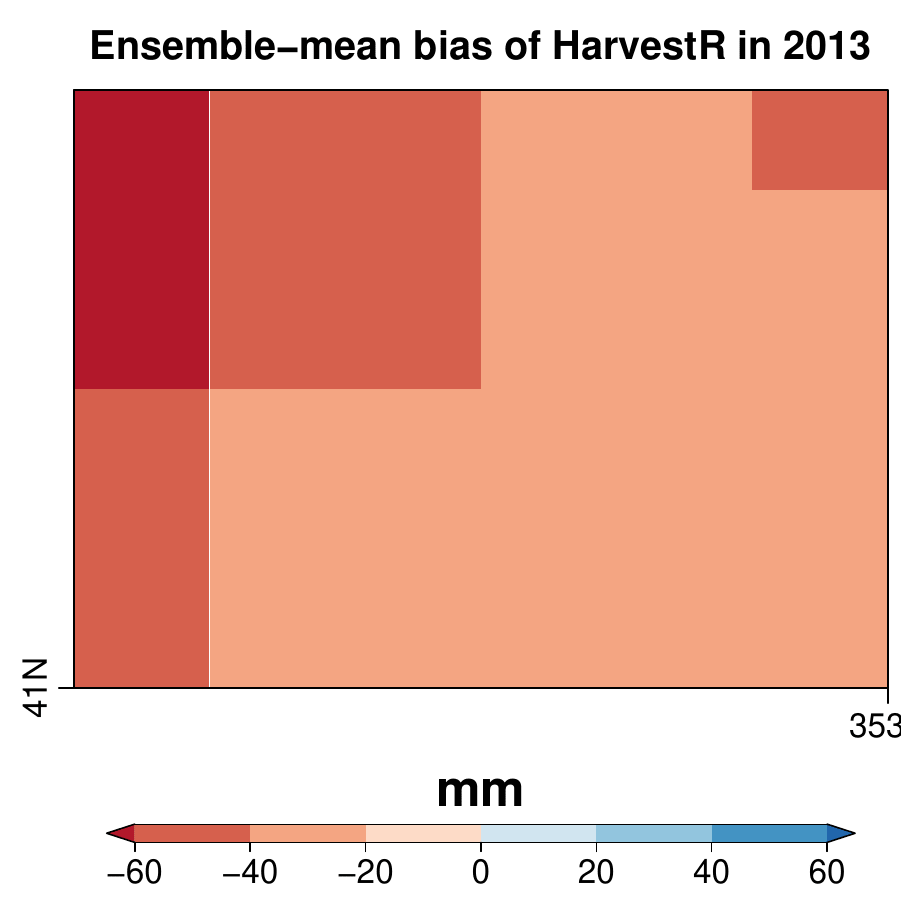In 2013, the ensemble-mean SEAS5 seasonal forecast of HarvestR is underestimated by up to 60 mm over Douro Valley region (the central four grid points).

### 2. PeriodMean

For the function `PeriodMean`, we use Growing Season Temperature (GST) as an example. GST is defined as the average of daily average temperatures between April 1st to October 31st in the Northern Hemisphere. It provides information onto which are the best suited varieties for a given site or, inversely, which are the best places to grow a specific variety. For existing vineyards, GST also informs on the suitability of its varieties for the climate of specific years, explaining quality and production variation. Many grapevine varieties across the world have been characterized in function of their GST optimum.

Firstly, we prepare a sample data of daily mean temperature of SEAS5 and ERA5 data sets with the same starting dates, spatial domain, interpolation grid and method by running

``````S5path <- list(path = '/esarchive/exp/ecmwf/system5c3s/\$STORE_FREQ\$_mean/\$VAR_NAME\$_f6h/\$VAR_NAME\$_\$YEAR\$\$MONTH\$01.nc')
ERA5path <- list(path = '/esarchive/recon/ecmwf/era5/\$STORE_FREQ\$_mean/\$VAR_NAME\$_f1h-r1440x721cds/\$VAR_NAME\$_\$YEAR\$\$MONTH\$.nc')

c(tas_exp, tas_obs) %<-% CST_Load(var = 'tas', exp = list(S5path), obs = list(ERA5path),
sdates = sdates, lonmax = 353, lonmin = 352.25,
latmax = 41.75, latmin = 41,
storefreq = 'daily',
nmember = 3, output = "lonlat",
grid = "r1440x721", method = 'bicubic')
``````

The output contains observations `tas_dv\$obs\$data` and forecast `tas_dv\$exp\$data`, and their dimensions and summaries are like

``````dim(tas_obs\$data)
#dataset  member   sdate   ftime     lat     lon
#      1       1       4     214       4       4

dim(tas_exp\$data)
#dataset  member   sdate   ftime     lat     lon
#      1       3       4     214       4       4

summary(tas_obs\$data - 273.15)
#  Min. 1st Qu.  Median    Mean 3rd Qu.    Max.
#  3.63   13.97   17.25   17.29   20.75   30.21

summary(tas_exp\$data - 273.15)
# Min. 1st Qu.  Median    Mean 3rd Qu.    Max.
# 0.54   11.65   16.56   16.50   21.25   31.41

``````

To compute the GST for both observation and forecast, run the following lines

``````# change the unit of temperature from °C to K

tas_exp\$data <- tas_exp\$data - 273.15
tas_obs\$data <- tas_obs\$data - 273.15

# compute GST

GST_exp <- CST_PeriodMean(tas_exp, start = list(1, 4), end = list(31, 10))
GST_obs <- CST_PeriodMean(tas_obs, start = list(1, 4), end = list(31, 10))

``````

Since the period considered for GST is the entire period for starting month of April, in this case the `start` and `end` parameters could be ignored.

The summaries and dimensions of the output are as follows:

``````summary(GST_exp\$data)
# Min. 1st Qu.  Median    Mean 3rd Qu.    Max.
# 14.23   15.78   16.50   16.50   17.17   18.70

summary(GST_obs\$data)
# Min. 1st Qu.  Median    Mean 3rd Qu.    Max.
# 15.34   16.77   17.22   17.29   18.00   18.75

dim(GST_exp\$data)
#dataset  member   sdate     lat     lon
#      1       3       4       4       4

dim(GST_obs\$data)
#dataset  member   sdate     lat     lon
#      1       1       4       4       4
``````

Here, we plot the 2013-2016 mean climatology of ERA5 GST by running

``````# compute ERA5 GST climatology
GST_Clim <- MeanDims(drop(GST_obs\$data), 'sdate')

cols <- c('#ffffd4','#fee391','#fec44f','#fe9929','#ec7014','#cc4c02','#8c2d04')
PlotEquiMap(GST_Clim, lon = tas_obs\$coords\$lon, lat = tas_obs\$coords\$lat,
intylat = 1, intxlon = 1, width = 6, height = 6,
filled.continents = FALSE, units = '°C', title_scale = .8,
axes_label_scale = 1, axes_tick_scale = 1, col_inf = cols,
margin_scale = c(1, 1, 1, 1), cols = cols[2:6], col_sup = cols,
brks = seq(16, 18.5, 0.5), colNA = 'white', bar_label_scale = 1.5,
toptitle = '2013-2016 mean ERA5 GST',
bar_extra_margin = c(0, 0, 0, 0), units_scale = 2)
``````

The ERA5 GST climatology is shown as below.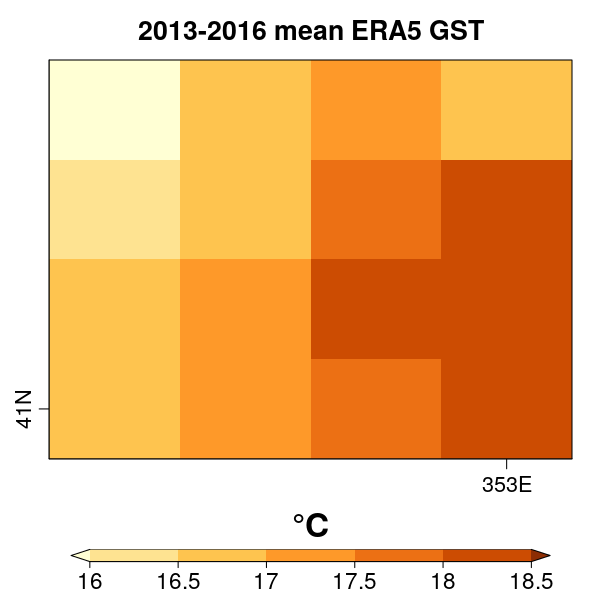ERA5 GST ranges from 17-18.5°C over the Douro Valley region for the period from 2013-2016 as shown in the figure.

### 3. TotalTimeExceedingThreshold

For the function `TotalTimeExceedingThreshold`, SU35 (Number of Heat Stress Days - 35°C) is taken as an example here. 35°C is the average established threshold for photosynthesis to occur in the grapevine. Above this temperature, the plant closes its stomata. If this situation occurs after veraison, maturation will be arrested for as long as the situation holds, decreasing sugar, polyphenol and aroma precursor levels, all essential for grape and wine quality. The higher the index, the lower will be the berry quality and aptitude to produce quality grapes.

SU35 is defined as the Total count of days when daily maximum temperatures exceed 35°C in the seven months into the future. There are three indicators sharing the similar definition as SU35: SU36, SU40 and Spr32. Their definition are listed as follows.

1. SU36: Total count of days when daily maximum temperatures exceed 36°C between June 21st and September 21st
2. SU40: Total count of days when daily maximum temperatures exceed 40°C between June 21st and September 21st
3. Spr32: Total count of days when daily maximum temperatures exceed 32°C between April 21st and June 21st

These indicators can be computed as well by using the function `TotalTimeExceedingThreshold` with different thresholds and periods indicated.

Here, we take SU35 as example, therefore the daily temperature maximum of the entire 7-month forecast period is needed for the computation of this indicator.

Load SEAS5 and ERA5 daily temperature maximum by running

``````S5path <- list(path = '/esarchive/exp/ecmwf/system5c3s/\$STORE_FREQ\$/\$VAR_NAME\$/\$VAR_NAME\$_\$YEAR\$\$MONTH\$01.nc')
ERA5path <- list(path = '/esarchive/recon/ecmwf/era5/\$STORE_FREQ\$/\$VAR_NAME\$-r1440x721cds/\$VAR_NAME\$_\$YEAR\$\$MONTH\$.nc')

c(tasmax_exp, tasmax_obs) %<-% CST_Load(var = 'tasmax', exp = list(S5path),
obs = list(ERA5path), sdates = sdates,
lonmax = 353, lonmin = 352.25,
latmax = 41.75, latmin = 41, storefreq = 'daily',
output = "lonlat", grid = "r1440x721",
method = 'bicubic', nprocs = 1)
``````

Check the unit of temperature to from °C to K for the comparison with the threshold defined (for example 35°C here).

``````tasmax_exp\$data <- tasmax_exp\$data - 273.15
tasmax_obs\$data <- tasmax_obs\$data - 273.15
``````

Computing SU35 for forecast and observation by running

``````threshold <- 35
SU35_exp <- CST_TotalTimeExceedingThreshold(tasmax_exp, threshold = threshold,
start = list(1, 4), end = list(31, 10))
SU35_obs <- CST_TotalTimeExceedingThreshold(tasmax_obs, threshold = threshold,
start = list(1, 4), end = list(31, 10))
``````

The summaries of SU35 forecasts and observations are given below.

``````summary(SU35_exp\$data)
# Min. 1st Qu.  Median    Mean 3rd Qu.    Max.
# 0.00    2.00    5.00    7.12   12.00   26.00

summary(SU35_obs\$data)
# Min. 1st Qu.  Median    Mean 3rd Qu.    Max.
# 0.000   0.000   1.000   2.609   5.000  10.000

``````

As shown in the summaries, SEAS5 SU35 forecasts are overestimated by 5 days in terms of mean value.

Therefore, `CST_BiasCorrection` is used to bias adjust the SU35 forecasts.

``````res <- CST_BiasCorrection(obs = SU35_obs, exp = SU35_exp)
SU35_exp_BC <- drop(res\$data)
summary(SU35_exp_BC)
#   Min. 1st Qu.  Median    Mean 3rd Qu.    Max.
# -1.419   0.000   1.613   2.831   4.756  17.768
``````

Since there are negative values after bias adjustment, all negative data is converted to zero.

``````SU35_exp_BC[SU35_exp_BC < 0] <- 0
summary(SU35_exp_BC)
#   Min. 1st Qu.  Median    Mean 3rd Qu.    Max.
#  0.000   0.000   1.613   2.943   4.756  17.768
``````

Plot the bias-adjusted SU35 forecast in 2016 by running

``````SU35_obs_Y2016 <- drop(SU35_obs\$data)[4, , ]
SU35_exp_Y2016 <- MeanDims(drop(SU35_exp\$data)[, 4, , ], 'member')
SU35_exp_BC_Y2016 <- MeanDims(SU35_exp_BC[, 4, , ], 'member')
cols <- c("#fee5d9", "#fcae91", "#fb6a4a", "#de2d26","#a50f15")

toptitle <- 'ERA5 SU35 forecast in 2016'
PlotEquiMap(SU35_obs_Y2016,
lon = tasmax_obs\$coords\$lon, lat = tasmax_obs\$coords\$lat,
intylat = 1, intxlon = 1, width = 6, height = 6,
filled.continents = FALSE, units = 'day', title_scale = .8,
axes_label_scale = 1, axes_tick_scale = 1, margin_scale = c(1, 1, 1, 1),
cols = cols[1:4], col_sup = cols, brks = seq(0, 8, 2),
toptitle = toptitle,
colNA = cols, bar_label_scale = 1.5,
bar_extra_margin = c(0, 0, 0, 0), units_scale = 2)

toptitle <- 'SU35 forecast in 2016'
PlotEquiMap(SU35_exp_Y2016,
lon = tasmax_obs\$coords\$lon, lat = tasmax_obs\$coords\$lat,
intylat = 1, intxlon = 1, width = 6, height = 6,
filled.continents = FALSE, units = 'day', title_scale = .8,
axes_label_scale = 1, axes_tick_scale = 1, margin_scale = c(1, 1, 1, 1),
cols = cols[1:4], col_sup = cols, brks = seq(0, 8, 2),
toptitle = toptitle,
colNA = cols, bar_label_scale = 1.5,
bar_extra_margin = c(0, 0, 0, 0), units_scale = 2)

toptitle <- 'Bias-adjusted SU35 forecast in 2016'
PlotEquiMap(SU35_exp_BC_Y2016,
lon = tasmax_obs\$coords\$lon, lat = tasmax_obs\$coords\$lat,
intylat = 1, intxlon = 1, width = 6, height = 6,
filled.continents = FALSE, units = 'day', title_scale = .8,
axes_label_scale = 1, axes_tick_scale = 1, margin_scale = c(1, 1, 1, 1),
cols = cols[1:4], col_sup = cols, brks = seq(0, 8, 2),
toptitle = toptitle,
colNA = cols, bar_label_scale = 1.5,
bar_extra_margin = c(0, 0, 0, 0), units_scale = 2)
``````

You can see the figure as below.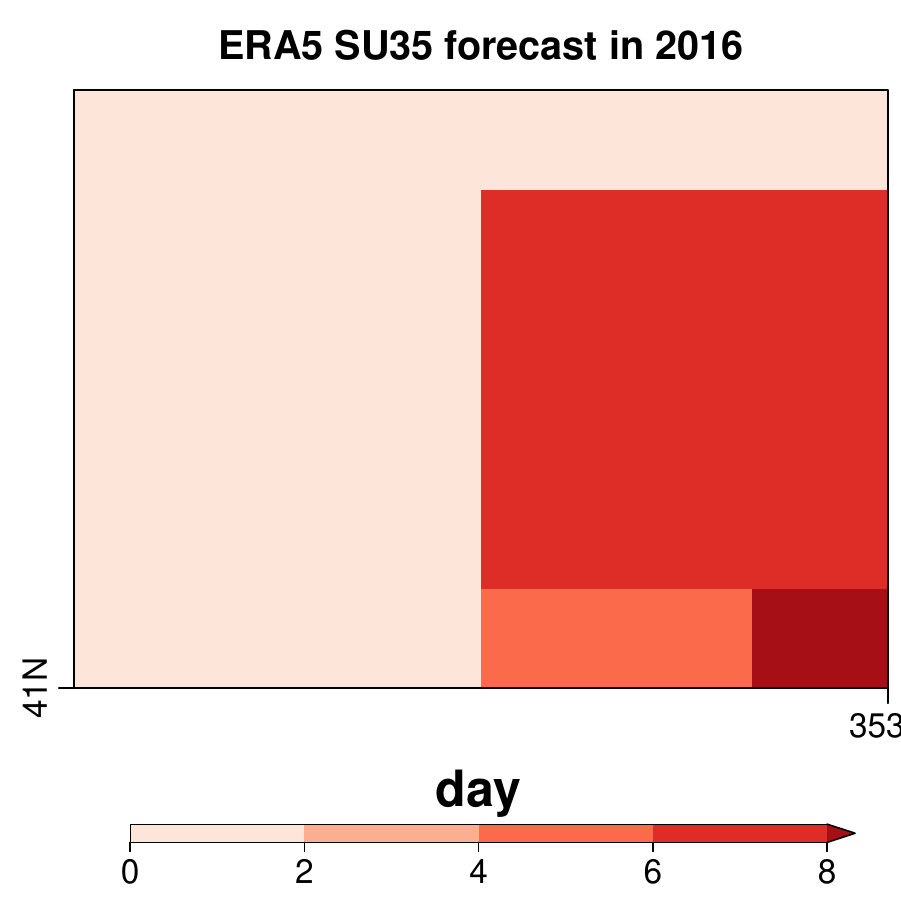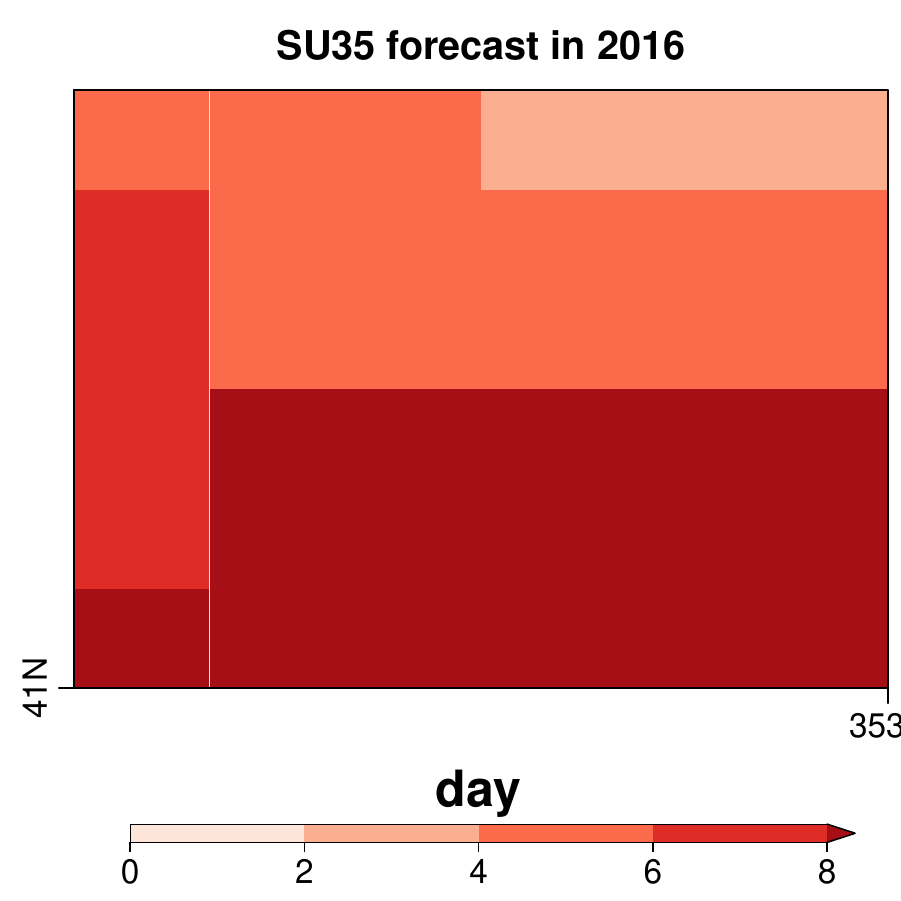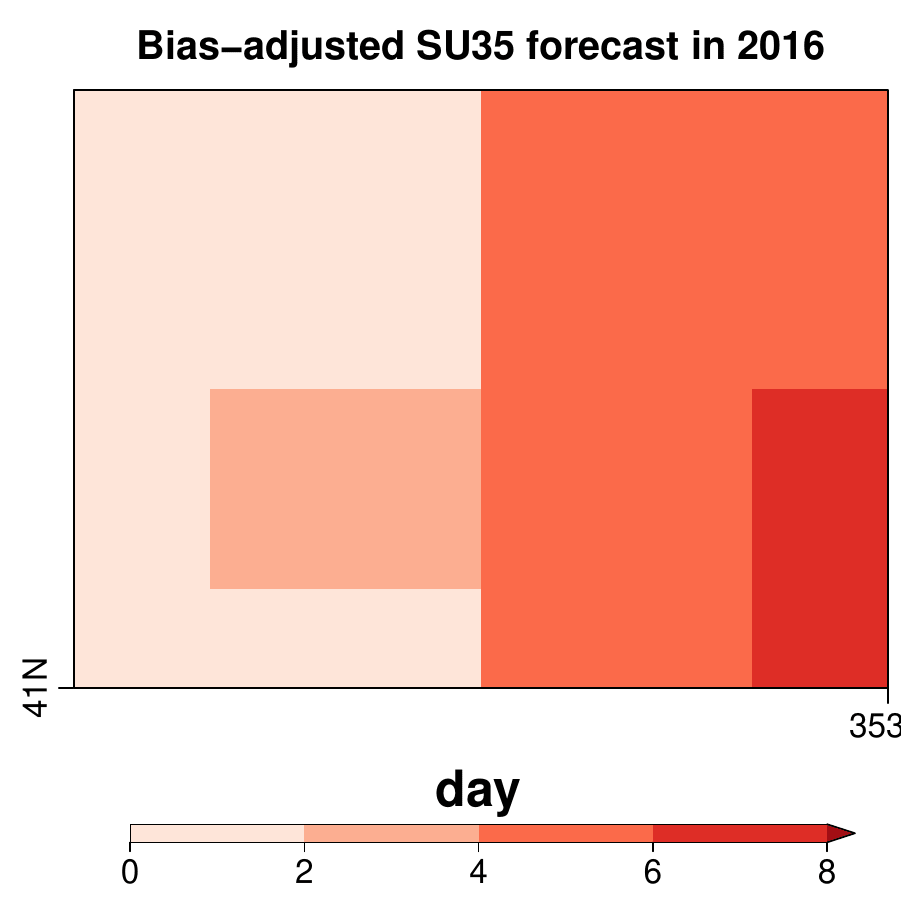As seen above, the bias-adjusted SU35 forecasts are much closer to the ERA5 results, although differences remain.

Beside of the original definition of SU35, here two supplementary functions in the package `CSIndicators` are demonstrated by computing its another definition with the percentile adjustment.

1. AbsToProbs -: to transform ensemble forecast into probabilities by using the Cumulative Distribution Function

## 2. QThreshold -: to transform an absolute threshold into probabilities.

The above two supplementary functions are required to compute SU35 with the percentile adjustment. The function `AbsToProbs` would be applied to forecast and the `QThreshold` would be used to convert the observations to its percentile based on the given threshold.

The revised definition of SU35 is to reduce the potential influence induced by the fixed threshold of temperature defined for the index, instead of using the absolute value, the percentile corresponding to 35°C for observation is compared to the percentile corresponding to the predicted daily maximum temperature before being considered as a ‘heat stress’ day.

As mentioned, the forecast is translated to its percentile by using the function `ABsToProbs` by running

``````exp_percentile <- AbsToProbs(tasmax_exp\$data)
S5txP <- aperm(drop(exp_percentile), c(2, 1, 3, 4, 5))
``````

After that, based on 35 of threshold, the percentile corresponding to each observational value can be calculated as follows.

``````obs_percentile <- QThreshold(tasmax_obs\$data, threshold = 35)
obs_percentile <- drop(obs_percentile)
``````

After translating both forecasts and observations into probabilities, the comparison can then be done by running

``````SU35_exp_Percentile <- TotalTimeExceedingThreshold(S5txP, threshold = obs_percentile,
time_dim = 'ftime')
``````

Compute the same ensemble-mean SU35 with percentile adjustment in 2016 by running

``````SU35_exp_per_Y2016 <- MeanDims(SU35_exp_Percentile[4, , , ], 'member')
``````

Plot the same map for comparison

``````toptitle <- 'SU35 forecast with percentile adjustment in 2016'
PlotEquiMap(SU35_exp_per_Y2016,
lon = tasmax_obs\$coords\$lon, lat = tasmax_obs\$coords\$lat,
intylat = 1, intxlon = 1, width = 6, height = 6,
filled.continents = FALSE, units = 'day', title_scale = .8,
axes_label_scale = 1, axes_tick_scale = 1, margin_scale = c(1, 1, 1, 1),
cols = cols[1:4], col_sup = cols, brks = seq(0, 8, 2),
toptitle = toptitle,
colNA = cols, bar_label_scale = 1.5,
bar_extra_margin = c(0, 0, 0, 0), units_scale = 2)

``````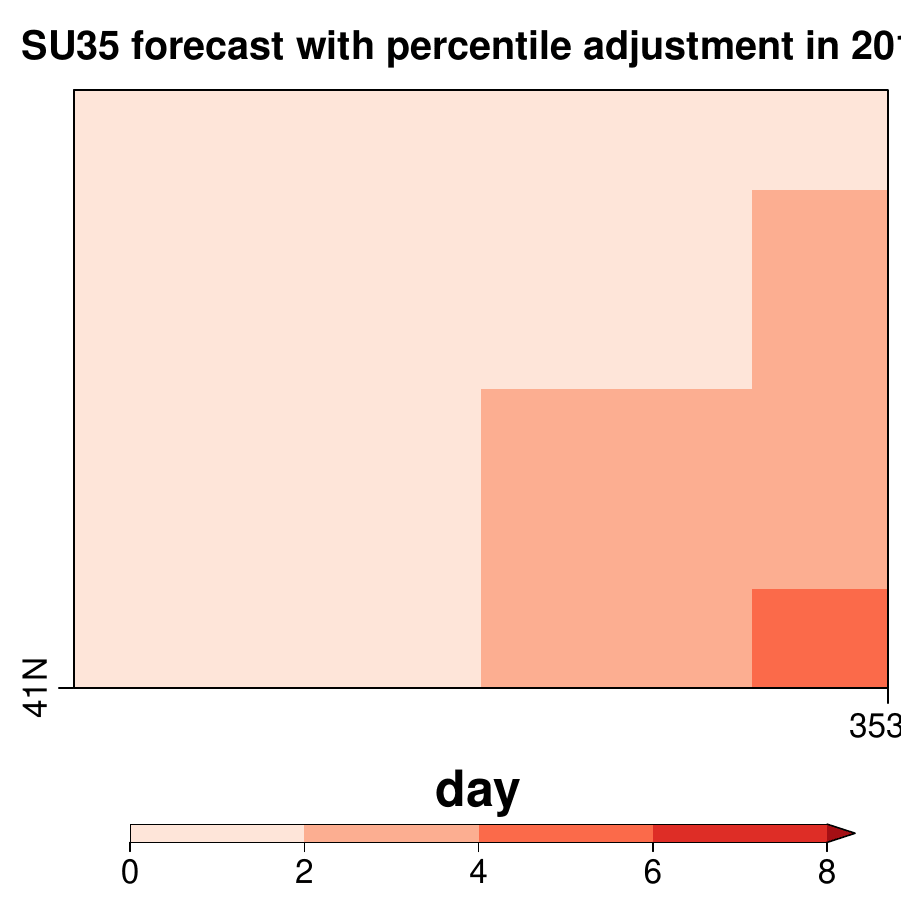As seen in the figure above, applying the percentile adjustment seems to implicitly adjust certain extent of bias which was observed in the non-bias-adjusted SEAS5 forecast.

The performance of comparison of skills between two definitions requires further analysis such as the application of more skill metrics.

### 4. AccumulationExceedingThreshold

The function ´AccumulationExceedingThreshold´ can compute GDD (Growing Degree Days).

The definition of GDD is the summation of daily differences between daily average temperatures and 10°C between April 1st and October 31st. Here, the tas (daily average temperature) used above (in Section 2. PeriodMean) is loaded again (Please re-use the section of loading tas in Section 2). As per the definition, `threshold` is set to 10 with `diff` set to TRUE so that the function will compute the differences between daily temperature and the threshold given before calculating summation.

Note: The data is in degrees Celsiusi at this point

``````GDD_exp <- CST_AccumulationExceedingThreshold(tas_exp, threshold = 10, diff = TRUE)
GDD_obs <- CST_AccumulationExceedingThreshold(tas_obs, threshold = 10, diff = TRUE)
``````

The summaries of GDD are

``````summary(GDD_exp\$data)
# Min. 1st Qu.  Median    Mean 3rd Qu.    Max.
# 1021    1331    1480    1469    1596    1873

summary(GDD_obs\$data)
# Min. 1st Qu.  Median    Mean 3rd Qu.    Max.
# 1195    1483    1569    1583    1730    1874
``````

To compute the correlation coefficient for the period from 2013-2016, run the following lines

``````# reorder the dimension
fcst <- Reorder(drop(GDD_exp\$data), c(4, 3, 2, 1))
obs <- Reorder(drop(GDD_obs\$data), c(3, 2, 1))

EnsCorr <- veriApply('EnsCorr', fcst = fcst, obs = obs, ensdim = 4, tdim = 3)
GDD_Corr <- Reorder(EnsCorr, c(2, 1))
``````

To plot the map of correlation coefficient of GDD for the 2013-2016 period.

``````cols <- c("#f7fcf5", "#e5f5e0", "#c7e9c0", "#a1d99b", "#74c476")
toptitle <- '2013-2016 correlation coefficient of GDD'
PlotEquiMap(GDD_Corr, lon = tas_obs\$coords\$lon, lat = tas_obs\$coords\$lat,
intylat = 1, intxlon = 1, width = 6, height = 6,
filled.continents = FALSE, units = 'correlation',
title_scale = .8, axes_label_scale = 1, axes_tick_scale = 1,
margin_scale = c(1, 1, 1, 1), cols = cols, brks = seq(0.5, 1, 0.1),
toptitle = toptitle, bar_label_scale = 1.5,
bar_extra_margin = c(0, 0, 0, 0), units_scale = 2)
``````

The map of correlation coefficient for the 2013-2016 period is shown as below.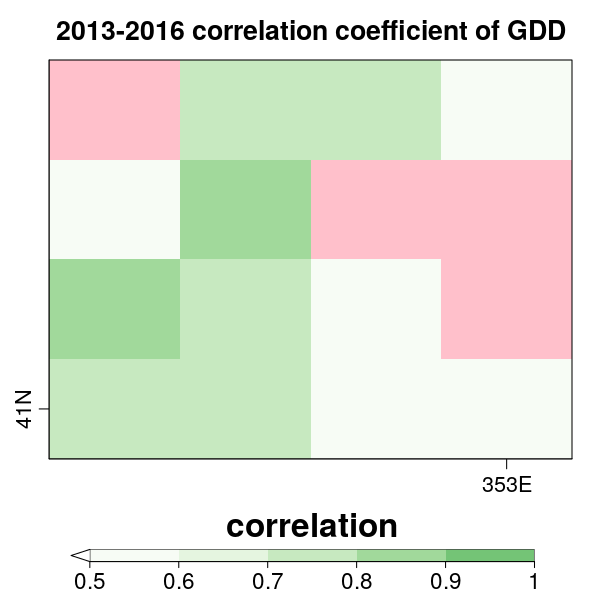The 2013-2016 correlation coefficients of the SEAS5 forecasts of GDD in reference with ERA5 reanalysis over Douro Valley range between 0.6 and 0.8.

### 5. TotalSpellTimeExceedingThreshold

One of the critical agricultural indicators related to dry spell is the Warm Spell Duration Index (WSDI), which is defined as the total count of days with at least 6 consecutive days when the daily maximum temperature exceeds its 90th percentile in the seven months into the future.

The maximum temperature data used in Section 3. Since the daily maximum temperature needs to compare to its 90th percentile, the function `Threshold` in the `CSIndicators` package is required to compute the percentile of observations used for each day. Here the same period (2013-2016) is considered.

``````tx_p <- CST_Threshold(tasmax_obs, threshold = 0.9)
``````

The output will be the 90th percentile of each day of each grid point derived by using all the years in the data.See the dimension and summary as below.

``````dim(tx_p\$data)
#dataset   ftime     lat     lon
#      1     214       4       4

summary(tx_p\$data)
# Min. 1st Qu.  Median    Mean 3rd Qu.    Max.
# 13.83   22.08   26.08   26.22   30.72   36.72
``````

With the prepared threshold (90th percentile), the WSDI can be computed by running

``````WSDI_exp <- CST_TotalSpellTimeExceedingThreshold(tasmax_exp, threshold = tx_p, spell = 6)
WSDI_obs <- CST_TotalSpellTimeExceedingThreshold(tasmax_obs, threshold = tx_p, spell = 6)
``````

After checking the summaries, compute the Fair Ranked Probability Skill Score (FRPSS) of WSDI by running the following lines

``````# Reorder the data
fcst <- Reorder(drop(WSDI_exp\$data), c(4, 3, 2, 1))
obs <- Reorder(drop(WSDI_obs\$data), c(3, 2, 1))

# summaries of WSDI
summary(fcst)
# Min. 1st Qu.  Median    Mean 3rd Qu.    Max.
# 0.00   13.00   28.00   31.22   46.00   82.00

summary(obs)
# Min. 1st Qu.  Median    Mean 3rd Qu.    Max.
# 9.00   19.00   22.50   23.16   26.00   33.00

# compute FRPSS
f <- veriApply('FairRpss', fcst = fcst, obs = obs, ensdim = 4, tdim = 3, prob = 1:2/3)\$skillscore
WSDI_FRPSS <- Reorder(f, c(2,1))
``````

Plot the map of WSDI FRPSS for the period from 2013-2016

``````cols <- c("#edf8fb", "#ccece6", "#99d8c9", "#66c2a4")
toptitle <- 'SEAS5 WSDI FRPSS (2013-2016)'

PlotEquiMap(WSDI_FRPSS, lon = tasmax_obs\$coords\$lon, lat = tasmax_obs\$coords\$lat,
intylat = 1, intxlon = 1, width = 6, height = 6,
filled.continents = FALSE, units = 'FRPSS', title_scale = .8,
axes_label_scale = 1, axes_tick_scale = 1, margin_scale = c(1, 1, 1, 1),
cols = cols[1:3], col_inf = 'white', col_sup = cols,
brks = seq(0, 0.9, 0.3), toptitle = toptitle, bar_label_scale = 1.5,
bar_extra_margin = c(0, 0, 0, 0), units_scale = 2)
``````

The FRPSS map for 2013-2016 SEAS WSDI is shown as below.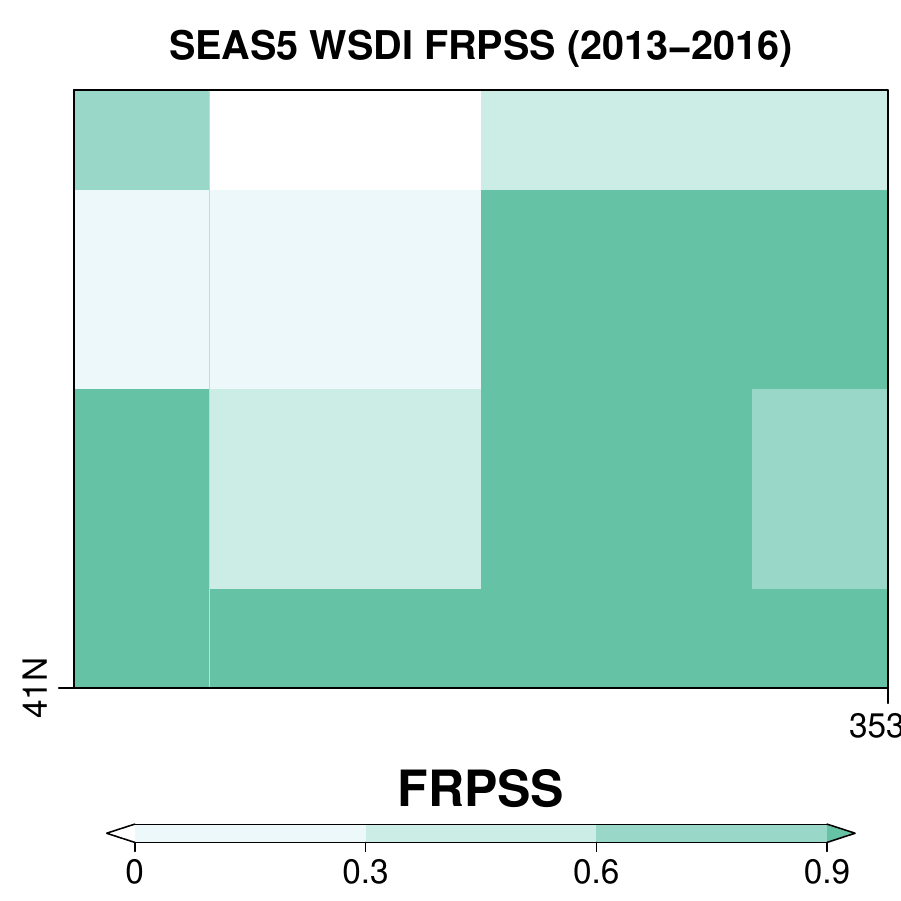As seen in the map, the FRPSS in the eastern part of Douro Valley falls in 0.6-0.9, which are good enough to be useful when compared to observational climatology.

In addition to the grape/wine sector focused here, the MEDGOLD project also works on the other two sectors: olive/olive oil and durum wheat/pasta. Furthermore, the climate services are also provided at the longer term (up to 30 years) by other project partners.